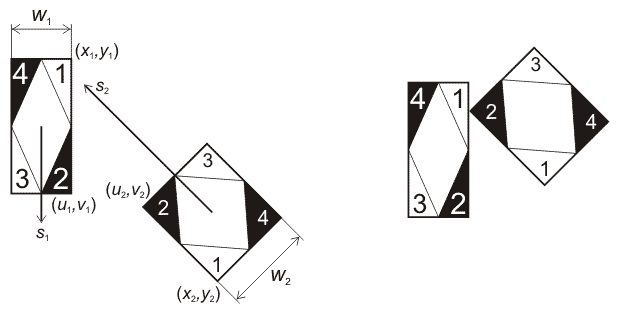시간 제한메모리 제한제출정답맞힌 사람정답 비율
1 초 128 MB0000.000%

## 문제

Two cars crashed on the road, receiving some damage each, and raising the usual question: "Who to blame?". To answer this, it is essential to thoroughly reconstruct the sequence of events. By gathering witness testimonies and analyzing tire tracks, positions and speeds of cars just before the impact were determined. From these positions until the crash the cars moved straight forward.

Your program must, given the available data, calculate for each car what part of it first came into contact with the other car. Parts are numbered as shown on the picture.## 입력

Input file contains twelve floating point numbers: $x_1, y_1, u_1, v_1, w_1, s_1, x_2, y_2, u_2, v_2, w_2, s_2$, where $(x, y)$ and $(u, v)$ -- coordinates of back-left and forward-left corners of the car, $w$ -- width of the car, $s$ -- speed of the car.

## 출력

Output file must contain two integers: $p_1, p_2$, where $p$ -- number of part which first contacted the other one (if two parts came into contact simultaneously, output the lesser of the part numbers).

## 제한

• $1 \le x_i, y_i, u_i, v_i, w_i \le 10^6, 0 \le s_i \le 10^6$.
• Input data is such that a crash certainly happens. Initially cars don't have common points.

## 예제 입력 1

1.0 2.0 10.0 2.0 1.0 10.0
50.0 1.0 40.0 1.0 1.0 20.0


## 예제 출력 1

2 2